## A positron with kinetic energy 1.60 keV is projected into a uniform magnetic field of magnitude 0.130 T, with its velocity vector making an

Question

A positron with kinetic energy 1.60 keV is projected into a uniform magnetic field of magnitude 0.130 T, with its velocity vector making an angle of 81.0° with the field. Find
(a) the period,
(b) the pitch p, and
(c) the radius r of its helical path.

in progress 0
2 months 2021-07-22T08:00:34+00:00 1 Answers 2 views 0

1. To find the given values we will first use the kinetic energy in order to find the speed. From the speed we can relate the term term the Pitch and the Radius. Below we will first present our given values and then perform the calculations: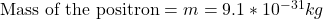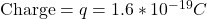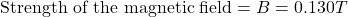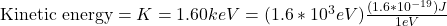=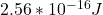Under the definition of Kinetic energy we have then,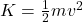Replacing with the values that we have to find the velocity,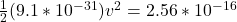Rearranging to find the velocity,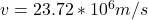PART A) The period is given as the relation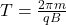Replacing with our values,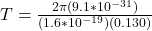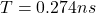PART B) The Pitch is given by,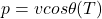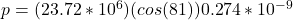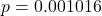PART C)  Now the radius is given under the equation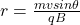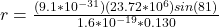r = 0.001025m